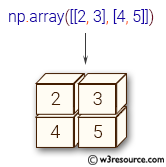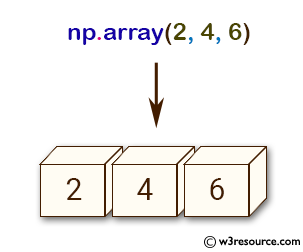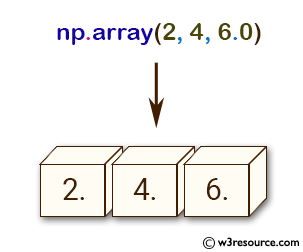# NumPy array creation: array() function

## array() function

The array() function is used to create an array.

Syntax:

`numpy.array(object, dtype=None, copy=True, order=’K’, subok=False, ndmin=0)`Version: 1.15.0

Parameter:

Name Description Required /
Optional
object An array, any object exposing the array interface, an object whose __array__ method returns an array, or any (nested) sequence. Required
dtype The desired data-type for the array. If not given, then the type will be determined as the minimum type required to hold the objects in the sequence. This argument can only be used to 'upcast' the array. For downcasting, use the .astype(t) method. optional
copy If true (default), then the object is copied. Otherwise, a copy will only be made if __array__ returns a copy, if obj is a nested sequence, or if a copy is needed to satisfy any of the other requirements (dtype, order, etc.). optional
order Specify the memory layout of the array. If object is not an array, the newly created array will be in C order (row major) unless ‘F’ is specified, in which case it will be in Fortran order (column major). If object is an array the following holds.
order nocopy copy=True
'K' unchanged F & C order preserved, otherwise most similar order
'A' unchanged F order if input is F and not C, otherwise C order
'C' C order C order
'F' F order F order

When copy=False and a copy is made for other reasons, the result is the same as if copy=True, with some exceptions for A, see the Notes section. The default order is ‘K’.

optional
subok If True, then sub-classes will be passed-through, otherwise the returned array will be forced to be a base-class array (default). optional
ndmin Specifies the minimum number of dimensions that the resulting array should have. Ones will be pre-pended to the shape as needed to meet this requirement optional

Return value:

[ndarray] An array object satisfying the specified requirements

Example -1: numpy.array(0 function

``````>>> import numpy as np
>>> np.array([2, 4, 6])
array([2, 4, 6])
>>> np.array([2, 4, 6.0])
array([ 2.,  4.,  6.])
>>> np.array([[2, 3], [4, 5]])
array([[2, 3],
[4, 5]])
``````

Pictorial Presentation:Example-2: numpy.ones_like() function

``````>>> import numpy as np
>>> a = np.array([(2,4),(5,6)], dtype=[('x', '<i4'), ('y','i4')])
>>> a['y']
array([4, 6], dtype=int32)
>>> a['x']
array([2, 5], dtype=int32)
``````

Example-3: numpy.ones_like() function

``````>>> import numpy as np
>>> np.array(np.mat('2, 4; 5, 6'))
array([[2, 4],
[5, 6]])
>>> np.array(np.mat('2 4; 6 8'))
array([[2, 4],
[6, 8]])
``````

Example-4: numpy.ones_like() function

``````>>> import numpy as np
>>> np.array([2, 4, 6], ndmin=4)
array([[[[2, 4, 6]]]])
``````

Example-4: numpy.ones_like() function

``````>>> import numpy as np
>>> np.array(np.mat('2 4; 6 8'), subok=True )
matrix([[2, 4],
[6, 8]])
>>> np.array(np.mat('2 4; 6 8'), subok=False)
array([[2, 4],
[6, 8]])
``````

Python - NumPy Code Editor:

Previous: full_like()
Next: asarray()

﻿